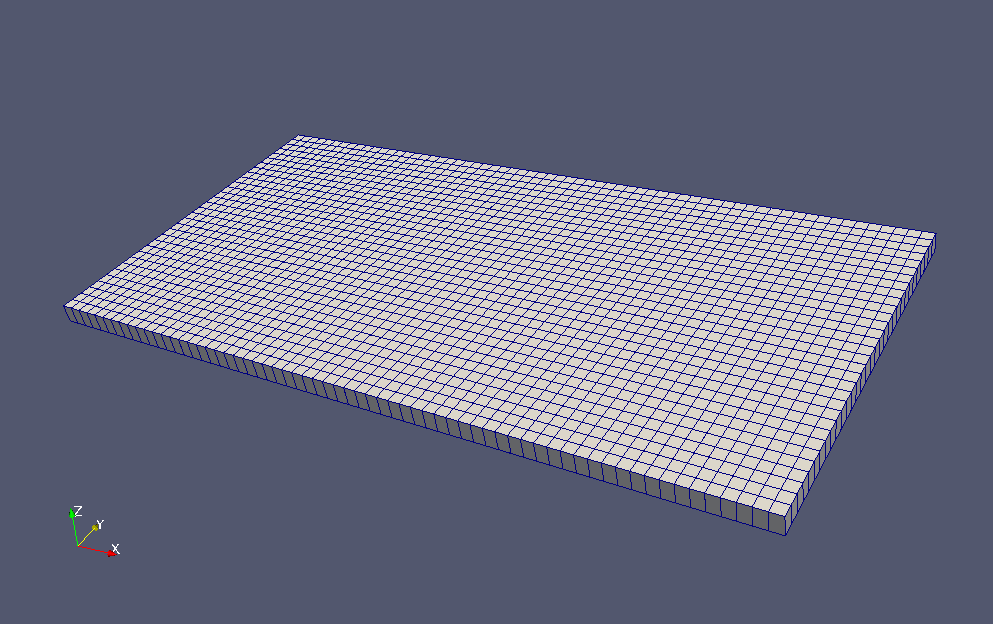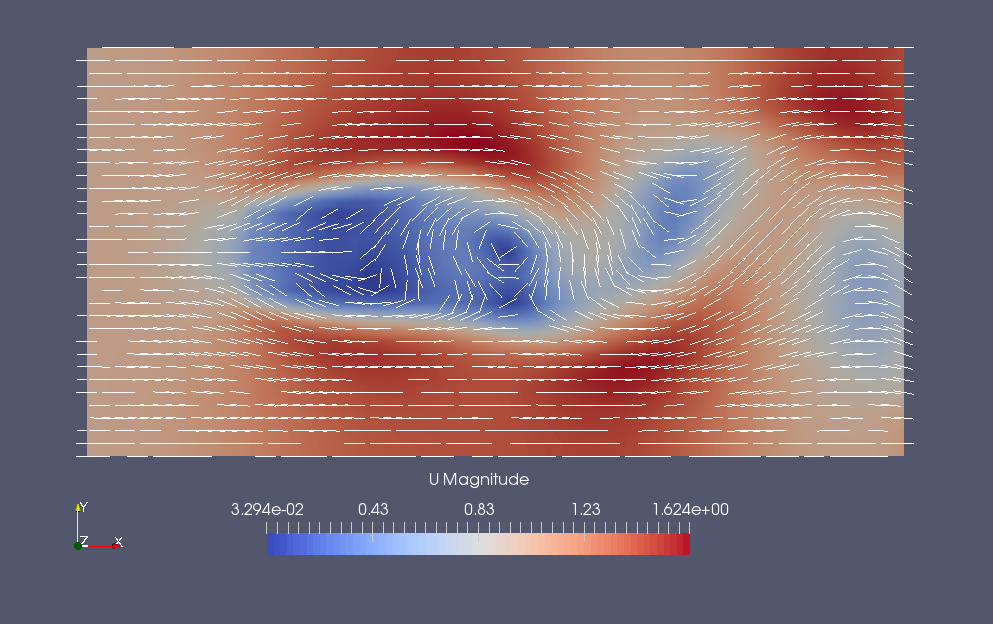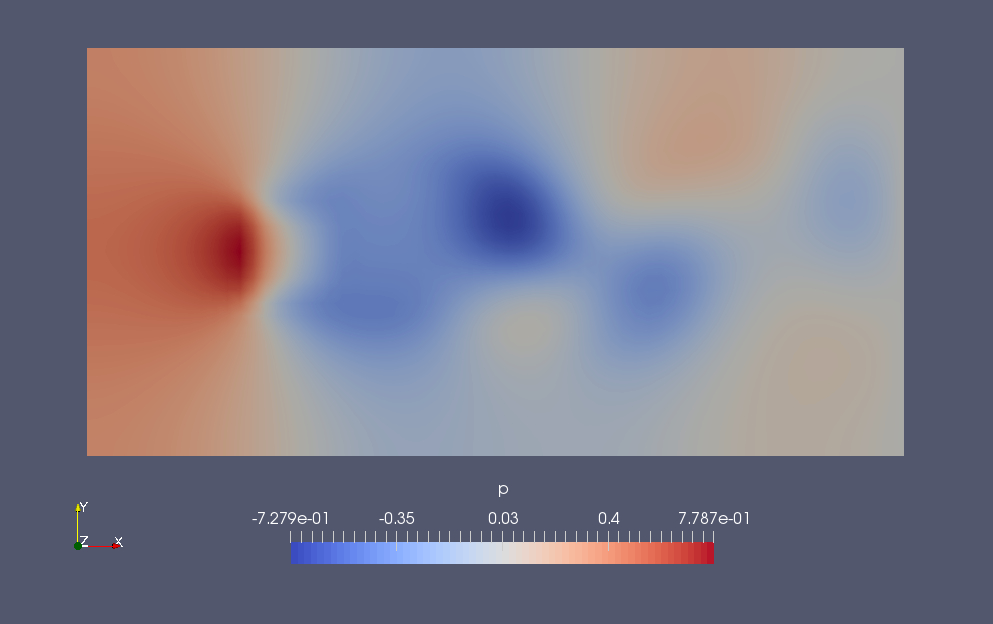﻿ Flow around an obstacle of a porous media - XSim

# Flow around an obstacle of a porous media

Update: June 1, 2017
OpenFOAM 4.x

$FOAM_TUTORIALS/incompressible/pisoFoam/laminar/porousBlockage ## Summary We calculate the flow around a porous body obstacle placed in a uniform flow from 0 sec to 100 sec. We solves the Navier-Stokes equations directly without using a turbulence model. The obstacle is defined as a cell zone "porousBlockage". The fluid flow in from region "inlet" at (1, 0, 0) m/s and out from region "outlet". A symmetry condition is set for the region symmetryPlane, which is the upper and lower surface in the Y direction. The problem will be solved as 2-dimensional problem with a single mesh in Z direction.Model geometry Porous media depends on the Darcy-Forchheimer law, and for a flow velocity ui (i=x, y, z) in direction i, a generation term Si (pressure drop) in the opposite direction of flow is added to the Navier-Stokes equations. Here, μ is the viscosity coefficient and ρ is the density. The parameters that determine the properties of the porous media, Dij, F, the direction of the properties, and the region in which the porous media, are specified in the file constant/fvOptions as follows. porosity1 { type explicitPorositySource; active yes; explicitPorositySourceCoeffs { selectionMode cellZone; cellZone porousBlockage; type DarcyForchheimer; D 1000; DarcyForchheimerCoeffs { d ($D $D$D);
f   (0 0 0);
coordinateSystem
{
type    cartesian;
origin  (0 0 0);
coordinateRotation
{
type    axesRotation;
e1  (1 0 0);
e2  (0 1 0);
}
}
}
}
}


The meshes are as follows.Meshes

The calculation result is as follows.

Flow velocity (U)Flow velocity at final time (U)Pressure at final time (p)

We can see that the flow is destabilized and a Kalman vortex is generated behind the obstacle.

## Commands

cp -r \$FOAM_TUTORIALS/incompressible/pisoFoam/laminar/porousBlockage porousBlockage
cd porousBlockage

blockMesh
topoSet

pisoFoam

paraFoam

## Calculation time

11.3 seconds *Single, Inter(R) Core(TM) i7-2600 CPU @ 3.40GHz 3.40GHz